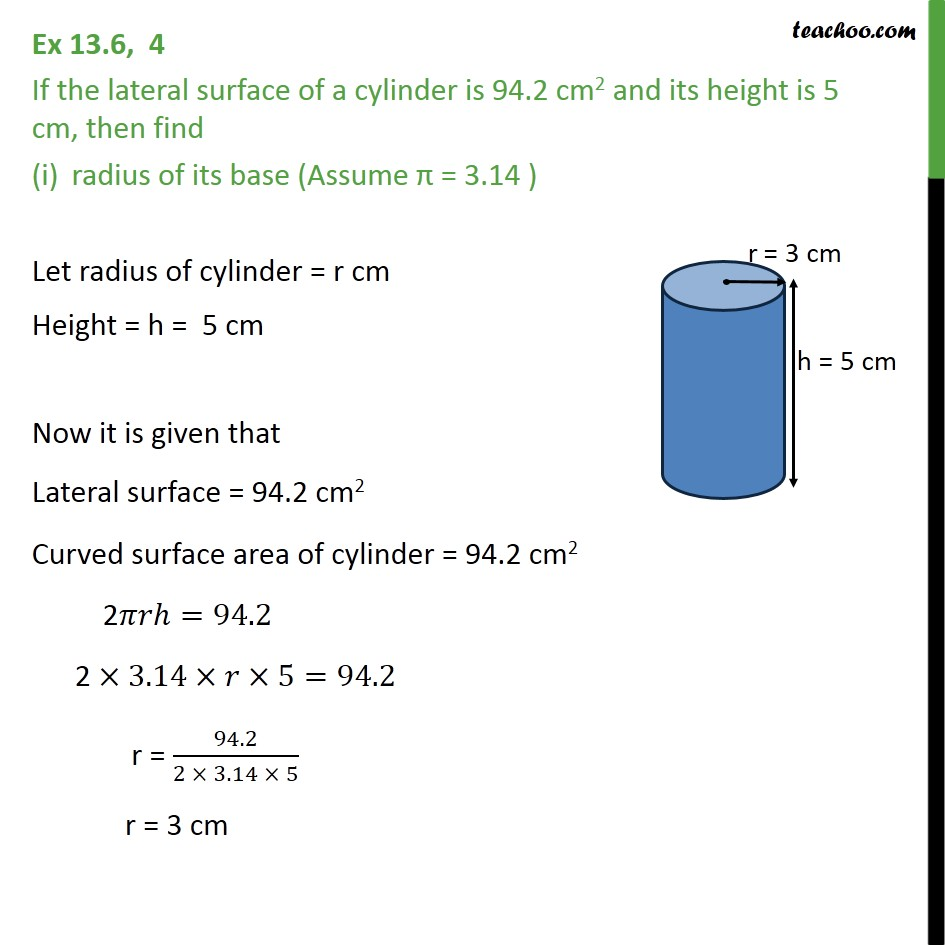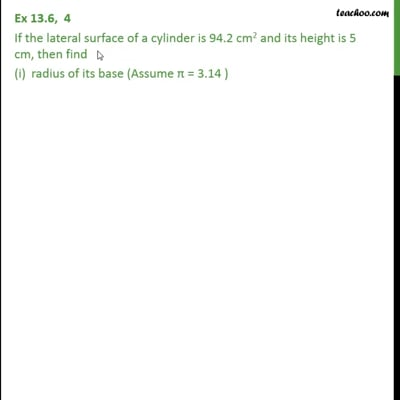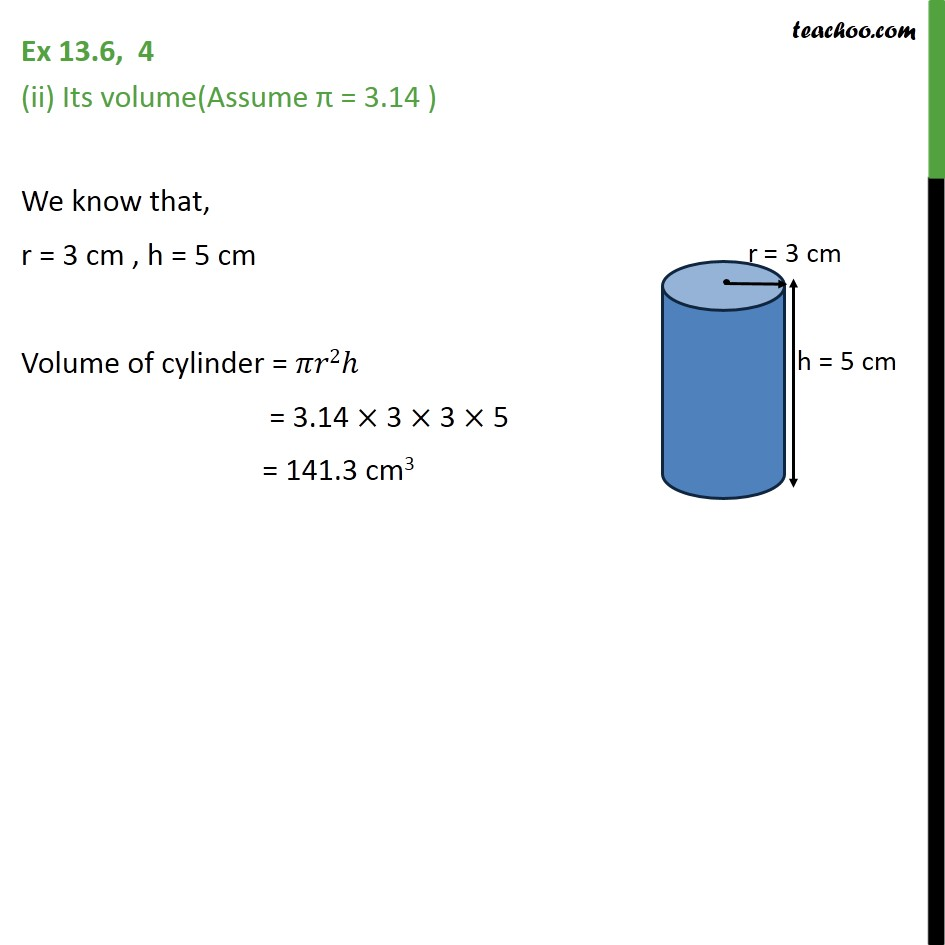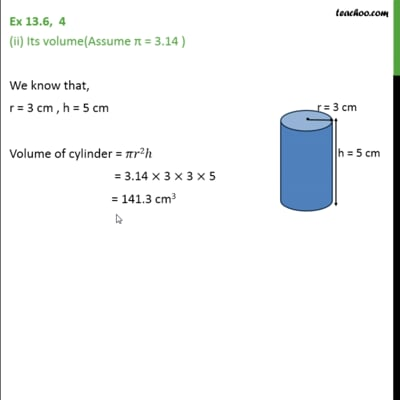Ex 13.6

Chapter 13 Class 9 Surface Areas and Volumes
Serial order wiseThis video is only available for Teachoo black usersThis video is only available for Teachoo black users

Solve all your doubts with Teachoo Black (new monthly pack available now!)

### Transcript

Ex 13.6, 4 If the lateral surface of a cylinder is 94.2 cm2 and its height is 5 cm, then find radius of its base (Assume π = 3.14 ) Let radius of cylinder = r cm Height = h = 5 cm Now it is given that Lateral surface = 94.2 cm2 Curved surface area of cylinder = 94.2 cm2 2𝜋𝑟ℎ=94.2 2 ×3.14×𝑟×5=94.2 r = 94.2/(2 × 3.14 × 5) r = 3 cm Ex 13.6, 4 (ii) Its volume(Assume π = 3.14 ) We know that, r = 3 cm , h = 5 cm Volume of cylinder = 𝜋𝑟2ℎ = 3.14 × 3 × 3 × 5 = 141.3 cm3